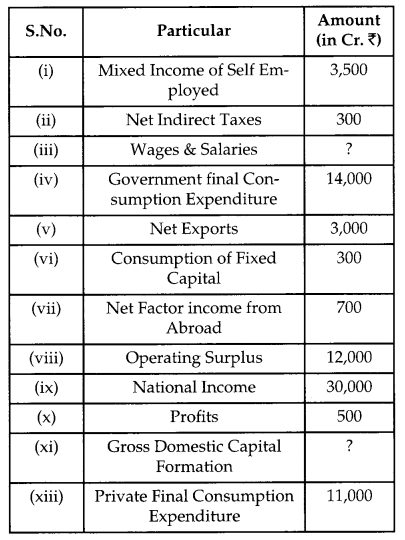## CBSE Previous Year Question Papers Class 12 Economics 2019 Delhi

Time allowed : 3 hours
Maximum marks: 80

General Instructions:

• All question in both sections are compulsory.
• Marks for questions are indicated against each questions.
• Question Nos. 1-4 and 13-16 are very short-answer questions carrying 1 marks each. They are required to be answered in one sentence each.
• Question Nos. 5-6 and 17-18 are short- answer questions carrying 3 marks each. Answer to them should normally not exceed 60 words each.
• Question No. 7-9 and 19-21 are also short-answer questions carrying 4 marks each. Answer to them should normally not exceed 70 words each.
• Question Nos. 10-12 and 22-24 are long- answer questions carrying 6 marks each. Answer to them should normally not exceed 100 words each.
• Answer should be brief and to the point and the above words limits should be adhered to as far as possible.

### CBSE Previous Year Question Papers Class 12 Economics 2019 Delhi Set – I

Section – A
(MICRO ECONOMICS)

Question 1.
In the given figure, the movement on the production possibility curve from point A to point B shows (Choose the correct alternative) 
(a) Growth of all the resources in the economy.
(b) Underutilisation of resources.
(c) Production of more units of Good X and less units of Good Y.
(d) Production of more units of Good Y and less unit of good X.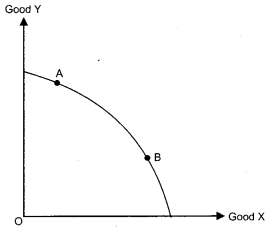(c) Production of more units of Good X and less units of Good Y.

Question 2.
Average fixed cost curve ……….. . (Choose the correct alternative) 
(a) is a straight line parallel to X-axis.
(b) is a straight line parallel to Y-axis.
(c) falls, as more units are produced.
(d) rises, as more units are produced.
OR
Which of the following formula is correct for calculating marginal cost? (Choose the correct alternative)
(a) MCN = TFCn — TFCN-1
(b) MCN = ACN — ACN-1
(c) MCN = AVCN — AVCn-1
(d) MCN = TCn — TCN-1
(c) Falls, as more units are produced.
OR
(d) MCN = TCn – TCN-1

Question 3.
The average product curve in the input-output plane, will be ______ (Choose the correct alternative) 
(a) as ‘S’ shaped curve
(b) an inverse ‘S’ shaped curve
(c) an ‘U’ shaped curve
(d) an inverse ‘U’ shaped curve
(d) an inverse ‘U’ shaped curve.

Question 4.
If the market supply of a commodity X changes due to improvement in technology, the market supply curve will ______. (Fill up the blank) 
OR
If the market supply of a commodity X changes due to rise in price of a factor input, the market supply curve will _______. (Fill up the blank)
If the market supply of a commodity X changes due to improvement in technology, the market supply curve will shift in rightward direction.
OR
If the market supply of a commodity X changes due to rise in price of a factor input, the market supply curve will shift in leftward direction.

Question 5.
Identify and discuss the nature of the following newspaper reports in terms of positive or normative economic analysis : 
(i) “India jumped 23 points in the World Bank’s ease of doing business index to 77th place, highest in 2 years.”- The Economic Times
(ii) “Government should further liberalise the business rules.” – The Economic Times
(i) The above statement comes under the positive economics since positive economics describes facts which can be verified by actual data and above statement can be easily verified by available data.
(ii) The statement comes under normative economics since it describes opinions and how the problems of economy should be solved. The above statement is like a solution to solve the aforesaid problem.

Question 6.
Distinguish between substitute goods and complementary goods, with examples. 
Distinguish between normal goods and inferior goods, with examples.

 Basis Substitute Goods Complementary Goods Meaning These are those goods which can be easily used in place of other or these are replace­able goods.                ‘ These are those goods which are demanded to­gether to satisfy a want or these goods cannot be replaced with one another.
 Types of demand Substitute goods are competitive in nature. Complementary goods are demanded jointly. Relation The demand of one good varies directly                   with the price of its substitute. The demand for one good varies indirectly         or inversely with the price of its complementary good. Examples Tea and coffee, coke and pepsi Car and petrol bread and butter

OR

 Normal Good Inferior Good Meaning It  refers to those goods whose demand increases with increase in income or vice- versa. It refers to those goods whose demand decreases with the increase in income or vice- versa. Relation with income There is direct relation between income and demand for a normal good. Thus income effect is positive. There is indirect or inverse relation between income and demand for an inferior good. Thus income effect is negative. Examples Pure milk, rice etc. Toned milk and coarse cereals etc.

Question 7.
Discuss briefly, using a hypothetical schedule, the relation between marginal utility and total utility. 
OR
Discuss briefly, using a hypothetical schedule, the concept of diminishing marginal rate of substitution.
The relationship between marginal utility and total utility can be explained with the help of following table:

 Units of consumption MU (Utils) TU (Utils) 1 10 10 2 8 18 3 6 24 4 4 28 5 2 30 6 0 30 7 -2 28

The following relationship is observed by the above schedule-
(i) TU increases as long as MU is more than zero, (upto 5 units of consumption)
(ii) When MU diminishes, TU increases at a diminishing rate.
(iii) TU is maximum where MU is zero. This happens when 6 units are consumed.
(iv) TU starts declining when MU becomes negative. It happens after 6 units of consumption.
OR
Concept of Diminishing Marginal rate of substitution:
(i) The assumption of diminishing marginal rate of substitution states that the consumer will be willing to sacrifice lesser units of good Y, so as to gain additional unit of good X.
(ii) Diminishing marginal rate of substitution is the reason behind convexity of indifference curve to the origin.
The following table shows different combination of good X and good Y which provide same level of satisfaction to the consumer.The above schedule shows that for each additional unit of good X, consumer is willing to sacrifice lesser and lesser units of good Y. For example, for 2nd unit of X, he is willing to sacrifice 6 units of Y. For 3rd unit of X, he is willing to sacrifice 5 units of Y and so on.

Question 8.
Complete the following cost schedule : 

 Quantity (in Units) 0 1 2 3 4 Total cost (in ₹) 200 …….. …….. …….. 490 Total variable cost (in ₹) 0 …….. 180 …….. …….. Average variable cost (in ₹) ___ 100 …….. 80 ……..

 Quantity 0 1 2 3 4 Total cost in (₹) 200 300 380 440 490 Total variable cost in (₹) 0 100 180 240 290 Average variable cost (₹) 90 80 72.5

Question 9.
In the given diagram, OP is the market determined price and OP1 is the price fixed by the government [1, 3]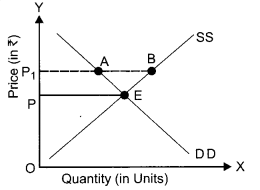(a) Identify whether the diagram represents, price ceiling or price flooring.
(b) Discuss the likely behaviour of the market in the given condition.
OR
Suppose the demand and supply equations of a commodity X in a perfectly competitive market are given by:
Qd = 1700 – 2P
Qs = 1300 + 3P
Calculate the value of equilibrium price and equilibrium quantity of the commodity X.
(a) The diagram represent the condition of price floor.

(b) The government fixes the prices of several goods higher than their equilibrium price to protect the interests of certain category of producers.
A price floor will only impact the market if it is greater than the free-market equilibrium price. If the floor price is greater than the economic price, the immediate result will be a surplus in supply. A price floor will also lead to a more inefficient market and a decreased total economic surplus. Economic surplus, is the sum of consumer and producer surplus. An effective price floor will raise the price of good hence decreased consumer surplus but increased surplus for producers.
OR
Qd = 1700 – 2P
Qs = 1300 + 3P
For calculating equilibrium price and quantity, put the above equation equal to each other or equilibrium is established
when Qd = Qs
1700 – 2P = 1300 + 3P
1700 – 1300 = 3P + 2P
400 = 5P ⇒
P = $$\frac{400}{5}$$ = 80
P = ₹ 80
Now put the value of price in any of the above equation
Qd = 1700 – 2P                       Qs= 1300 + 3P
= 1700 – 2 × 80      Or                =1300 + 3 × 80
= 1700 – 160                                = 1300 + 240
= 1540                                          = 1540
So equilibrium price = ₹ 80
Equilibrium quantity = 1540 units.

Question 10.
(a) Define price elasticity of demand.
(b) If the price of a commodity rises by 40% and its quantity demanded falls from 150 units to 120 units, calculate coefficient of price elasticity of demand for the commodity. [2, 4]
(a) Price elasticity of demand is a measure of degree of responsiveness of the demand for a good to change in its price or it is defined as the percentage change in demand for the good divided by the percentage change in its price.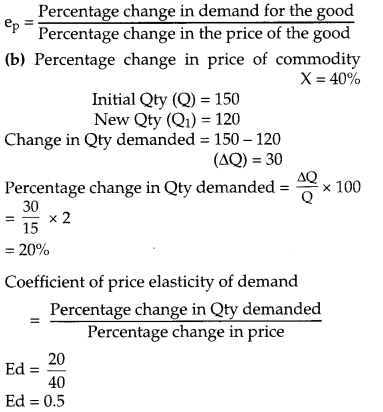Question 11.
What is meant by “diminishing returns to a factor”? Discuss any two reasons for the operation of diminishing returns to a factor. 
The law of diminishing returns to a factor states that when additional units of a variable factor are applied, other factors are remaining constant, the total product increases at a diminishing rate or marginal product diminishes.
Two main reasons for the operation of diminishing returns to a factor are:

• Over-utilisation of the fixed factor: As we keep on increasing the variable factor along with the fixed factor eventually a position comes when the fixed factor has its limits and starts yielding diminishing return.
• Improper coordination between Fixed and Variable factors: After a certain level of employment, the production process becomes too crowded with variable input and factor proportion tends to become less and less suitable for the production.

Question 12.
Elaborate three features of monopoly form of market. 
OR
Distinguish between perfect competition and monopolistic competition on the basis of following:
(a) Number of sellers
(b) Nature of product
(c) Selling cost
Monopoly is a market situation in which there is a single seller, with no close substitutes for commodity, it produces and there are barriers to entry. Three main features of monopoly form of market are :
(1) Single seller: There is a single producer of a commodity therefore the difference between firm and industry disappears, the firm has full control over supply of the commodity.
(2) No close substitutes: The product offered by a monopolist has no close substitute. So, the monopoly firm has no fear of competition from new or existing rivals.
(3) Restriction on entry: There exist strong barriers to entry of new firm under monopoly. As a result, a monopoly firm can manipulate the market and earn abnormal profits in the long run too.
OR
Distinction between perfect competition and monopolistic competition.

 Basis Perfect Competition Monopolistic Competition 1. Number of sell­ers There are large number of sell­ers. There are also a large number of sellers but less than perfect com­petition. 2. Nature of prod­uct Homogenous pr­oducts are sold under this mar­ket situation. Products are dif­ferent but are close substitutes of one another or product differen­tiations is there. 3. Selling There is no scope for selling cost Huge amount money is spent on advertisement etc.,

Section -B
(MACRO ECONOMICS)

Question 13.
Give any two examples of flow concept. 
Two examples of flow concept are National income, Investment.

Question 14.
Define the term ‘tax’. 
Tax is the compulsory financial charge imposed on an individual through government.

Question 15.
Suppose in a hypothetical economy, the income rises from ₹ 5,000 crores to ₹ 6,000 crores. As a result, the consumption expenditure rises from ₹ 4,000 crores to ₹ 4,600 crores. Marginal propensity to consume in such a case would be . (Choose the correct alternative) 
(a) 0.8
(b) 0.4
(c) 0.2
(d) 0.6
(d)
Initial income = ₹ 5,000 crores
New income = ₹ 6,000 crores
Change in income (ΔY) = 6000 – 5000 = ₹ 1,000 crore
Initial consumption = ₹ 4,000 crore
New consumption = ₹ 4,600 crore
Change in consumption = 4600 – 4000 (ΔC) = ₹ 600 crore
Marginal propensity to consume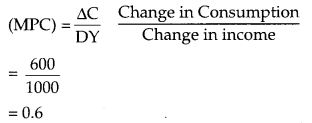Question 16.
What is a meant by primary deficit? 
OR
What is meant by fiscal deficit?
Primary deficit is the difference between fiscal deficit and interest payments, made by goverment.
Primary deficit = Fiscal deficit – Interest payments
OR
Fiscal deficit refers to the excess of total expenditure over the sum of revenue receipts and total capital receipts excluding borrowings.

Question 17.
Define the problem of double counting in the computation of national income. State any two approaches to correct the problem of double counting.  
OR
“Gross Domestic Product (GDP) does not give us a clear indication of economic welfare of a country.” Defend or refute the given statement with valid reason.
Double Counting – Double counting means counting of the value of the same product more than once in calculating the national income.
Two ways of avoiding double counting :

• Take the value of final goods only: According to this method, the value of intermediate goods is not considered. Only the value of final goods should be added to determine the national income.
• Adopt value added method: According to this method, sum total of the value added by each production unit should be taken in the computation of national income.

OR
The given statement is completely true that GDP does not give us a clear indication of economic welfare of a country. GDP is a measure of economy’s production or it can be considered a component of welfare. A higher GDP means more production of goods and services in an economy during a given year. Therefore, a higher GDP also means that more goods and services were available to the people of the country during the year. But it does not indicate that the people were better off during the year. In other words, a higher GDP may not necessarily mean higher welfare of the people.

Question 18.
If in an economy :
Change in initial investments (AI) = ₹ 500 crores
Marginal Propensity to Save (MPS) = 0.2 
Find the value of the following :
(a) Investment multiplier (k)
(b) Change in final income (ΔY)
Change in initial investment (ΔI) = ₹ 500 crores
Marginal propensity to save (MPS) = 0.2Question 19.
How are capital receipts different from revenue receipts ? Discuss briefly.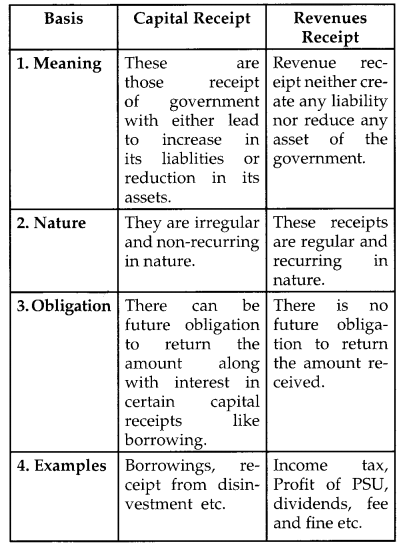Question 20.
State and discuss the components of Aggregate Demand in a two sector economy. 
OR
In the given figure, what does the gap “KT” represent? State any two fiscal measures to correct the situations.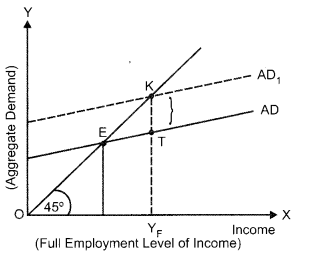Aggregate demand refers to demand for all goods and services in the economy during a given period of time. The components of Aggregate demand in a two sector econmony are: .
(i) Consumption Expenditure (c): It is that portion of the income which is spend on purchase of goods and services by the consumers in an economy during the accounting period.
(ii) Investment Expenditure (I):  The addition to the stock of physical capital and change in 1 inventories of a firm in an economy.
OR
The vertical gap ‘K T’ represents ‘Deficient Demand.
The fiscal measures to correct deficient demand I are:
(i) Reduction in taxes— The revenue Policy of the government is expressed in term of taxes. During deflation the government will impose the lower amount of taxes so that the purchasing power of people be increase. To control deficient demand government have to increase liquidity ‘ in the economy.
(ii) Increase in government expenditure: Government has to invest huge amount on the public works like roads, buildings, etc. thereby increasing the money income of the people and their demand for goods and services.

Question 21.
Discuss the working of the adjustment mechanism in the following situations : 
(a) Aggregate demand is greater than Aggregate supply.
(b) Ex Ante Investment are lesser than Ex Ante Savings.
(a) Aggregate demand is greater than Aggregate supply:
When AD> AS, it means that buyers are planning, to buy more goods and services than producers are planning to produce. As a result, inventories start falling and come below the desired level. To bring back the inventories at the desired level producers expand production. This raises the income level which keeps on rising till the AD and AS once again become equal.

(b) Ex-Ante Investments are lesser than Ex-Ante Savings:
When planned savings are more than planned investment, it means that household are not consuming as much as firms expected them to do so. This will lead to build up of undesired inventory. To clear the undesired rise in inventory, firms reduces its production, which also means fall in income and hence saving reduces till planned savings and planned investments are equal.

Question 22.
(a) Define “Trade surplus”. How is it different from “Current account surplus”? [3,3]
(b) “Indian Rupee (₹) plunged to all time low of ₹ 74.48 against the US Dollar ($)”. -The Economic Times In the light of the above report, discuss the , impact of the situation on Indian Imports. Answer: (a) Trade surplus refers to excess of value of exports of visible items over the value of import of visible items in the balance of payment account of a country. Current account surplus refers to excess of receipts from value of export of visible items, invisible items and unilateral transfers over payment of value of import of visible items, invisible items and unilateral transfers. It is a broader concept as compared to trade surplus. (b) “Indian rupee (₹) plunged to all time low of ₹ 74.48 against the US Dollar ($)”
The above statement indicates the depreciation of Indian currency against the foreign currency dollar ($). Depreciation means the fall in the value of rupee in terms of foreign currency. More rupees are now required to buy a unit of foreign currency. This will make foreign goods costlier to India. As a result, imports are likely to fall. Question 23. (a) State any two components of Mi measure of money supply. [2,4] (b) Elaborate any two instruments of Credit Control, as exercised by the Reserve Bank of India. OR Define Credit Multiplier. What role does it play in determining the credit creation power of the banking system? Use a numerical illustration to explain.  Answer: (a) Two components of M1 measure of money supply: M1 = C + OD + DD Where C = Currency held by the public OD = Other deposits DD = Demand deposits held by commercial banks OD is the other deposits with the RBI. These are the demand deposits held by the RBI of all economic units except the government and banks. It includes demand deposits of public financial institutions (like IDBI, etc.), foreign Central Banks and government, IMF, World Bank etc. DD is demand deposits which means those deposits which can be withdrawn at any time by the account holders. Current account deposits are included is demand deposits. (b) Two instruments of Credit Control are— (i) Bank Rate Policy : The bank rate is the rate at which the Central Bank lends money to commercial banks. Through changes in bank rate, the Central Bank affects the money supply in the economy. When credit is to be expanded the Central Bank reduces the bank rate. A low bank rate encourages the banks to keep small proportion of their deposits as reserves, since borrowing from Central Bank is now less costly than before. As a result banks use greater proportion of their funds for giving at loan to borrowers or investors. Thus, money supply increases. The bank rate is lowered during deflation. The reverse occurs during inflation and RBI control credit in the economy. (ii) Open Market Operations-It refers to the buying and selling of government securities by the Central Bank from/to the public and banks. When Central Bank buys government securities, it adds to cash balances of the economy. If cash balances are increased in the economy, there will be more deposits with commercial banks and hence, more flow of credit and when Central Bank sells government securities it withdraws cash balances from the economy. When cash balances are reduced deposits with commercial banks decreases hence, flow of credit will decrease. OR Credit multiplier measures the amount of money that the banks are able to create in the form of deposits with every initial deposits. The credit creation of the commercial bank depends on credit mutiplier as it is inversly related to LRR. Higher the credit multiplier, higher will be the total credit created and vice versa. Numerical example: Suppose the amount of initial deposit is ₹ 1000 and LRR is 10%. The banks will keep 10% i.e. ₹ 100 as reserve and lend the remaining ₹ 900 to borrowers. The borrowers will spend this money. It is assumed that ₹ 900 comes back to the banks. Bank again keep 10% ₹ 900, i.e. ₹ 90 as reserve and lend ₹ 810. This will further raise, the amount of deposits with the banks. In this way, deposits go on increasing number of times and the total deposit will be determined by the money multiplier. Money multiplier = $$\frac{1}{\mathrm{LRR}}=\frac{1}{0.10}$$ =10 The total deposit will be = Initial deposit × money multiplier = 1000 × 10 = ₹ 10,000 Question 24. Given the following data, find the missing value of Government Final Consumption Expenditure’ and Mixed Income of Self EmployedAnswer : Mixed income of self employed = (i) – [(viii) + (xii) + (v)] = 71,000 – [15,000 + 30,000 + 1000] = ₹ 2,500 crores Government final consumption expenditure = (i) – [(x) + (ii) + (v) + (ix)] + (vi) + (xi) = 71,000 – (40,000 + 10,000 + 1000 + 5000] + 2,000 + 3000 = ₹ 20,000 crores. ### CBSE Previous Year Question Papers Class 12 Economics 2019 Delhi Set – II Note: Except for the following questions, all the remaining questions have been asked in previous sets. Question 6. Good X and Good Y are substitute goods. If price of Good X increases, discuss briefly its likely impact on the demand for Good Y.  OR If the income of a consumer increases, discuss briefly its likely impact on the demand for an inferior good, Good X. Answer: If the good X and Y are substitutes, a rise in price of X will result in a rightward shifting in demand curve of Y because now good Y become cheaper in comparison of good X. As a result, demand for good Y will increase and consumer will substitute good Y over good X. OR As income increases the demand for an inferior good falls. The consumer buys less of inferior goods and prefer to buy superior substitutes. Hence there will be leftward shifting of demand curve in case of inferior goods.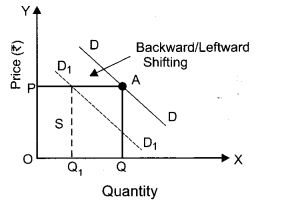Question 9. Explain the law of diminishing marginal utility using a hypothetical schedule.  OR What is a budget line? Why the budget line is left to right downward sloping? Answer: Law of Diminishing Marginal Utility- Law of Diminishing Marginal Utility states that keeping other factors constant, as more and more units of a commodity are consumed, the additional utility that we derive from it goes on declining. Numerical example:  Units of Orange Total Utility (Utils) Marginal Utility (Utils) 1 10 10 2 18 8 3 24 6 4 28 4 5 30 2 6 30 0 7 28 -2 8 24 -4 It is clear from the above table that the consumer gets marginal utility equal to 10 utils from the 1st unit of orange, 8 utils from 2nd, 6 utils from 3rd and so on. The marginal utility falls because of decline in the intensity of desire. A stage comes when marginal utility become zero. This is called the Point of satiety. At this point TU is maximum. If the consumer goes beyond the point, utility becomes negative. OR Budget line : Budget line is a locus of different combination of two goods, which the consumer consumes and which cost the consumer exactly equal to his income. Let us consider, a consumer who has only a fixed amount of money equal to ₹ 50 to spend. Further suppose that he spends his money income on two commodities X and Y, whose market prices are ₹ 10 per unit and ₹ 5 per unit respectively.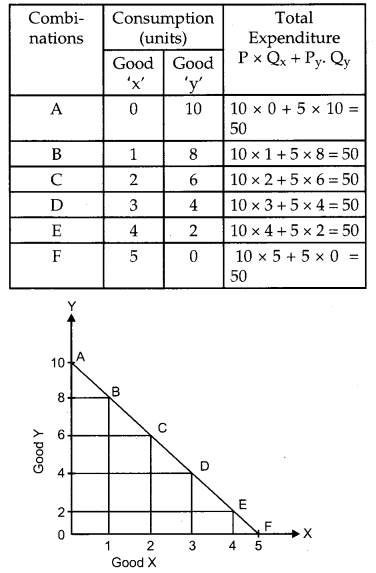By the above schedule and diagram that total expenditure remains same hence to purchase more of another commodity consumer needs to sacrifice other so the budget line moves from left to right or downward sloping. Question 11. Elaborate three main features of oligopoly form of market.  OR Distinguish between monopoly and monopolistic competition on the basis of the following: (a) Number of Sellers (b) Name of the Products (c) Selling cost Answer: Oligopoly Market- Oligopoly is a market where there are few large firms competing with each other. The competing firms are few in number but each one is large enough so as to be able to control the total industrial output. Three main features are : (1) Number of seller or few firms-The number of firms are very less in a oligopoly. By small number means that every seller controls a large share of total market supply and by his activities, he influences other seller’s activities and price of the commodity. (2) Interdependence – It is another important feature of oligopoly market situation. There is interdependence of the firms in decision making. This is because when the number of competitors is few, any change in price, output, product etc. by a firm will have a direct effect on its rival firm. (3) Nature of the Product – The product in such market situation may be homogenous or heterogeneous. If it is homogeneous then it is perfect oligopoly or pure oligopoly if there is product differentiation it is imperfect oligopoly. (4) Selling cost-Huge selling cost is applied under this market situation to attract the customers to the product. OR  Basis Monopoly Monopolistic Competition Number of sellers There is only a single seller in the market hence covering the whole market for its product. There exists large number of sellers in the market hence every seller covers a specific portion of market for its product. Nature of the product The product is unique with no close substitute. There exists prod­uct differentiation and each product has it’s close sub­stitute. Selling cost Monopoly firms need not to spend on the advertisements etc. since their product is unique. Huge amount of money is spent on the advertisement to promote the sale of the product. Section – B (MACROECONOMICS) Question 17. If in an economy: Change in initial investment (ΔI) = ₹ 700 crores Marginal Propensity to Save (MPS) = 0.2 Find the values of the following : (a) Investment Multiplier (k) (b) Change in final income (ΔY) Answer: (a) Change in initial investment (ΔI) = ₹ 700 crores Marginal propensity to save (MPS) = 0.2 Investment multiplier (k) = $$\frac{1}{\mathrm{MPS}}$$ = $$\frac{10}{2}$$ K = 5 (b) Change in final income (ΔY) K = $$\frac{\Delta \mathrm{Y}}{\Delta \mathrm{I}}$$ ΔY = K × ΔI = 5 × 700 = ₹ 3500 crores Question 20. How are capital expenditure different from Revenue expenditore ? Discuss briefly. Answer: Revenue expenditure is that expenditure of the government that neither creates any asset nor reduces any liability. For example, expenditure on salaries, Pensions, interestpaymentetc. whereas capital expenditure is that expenditure of the government that either creates assets or reduces liabilities. For example expenditure on construction of roads, repayment of loans etc. Question 23. Given the following data, find the missing value of ‘Private Final Consumption Expenditure and ‘Operating Surplus’.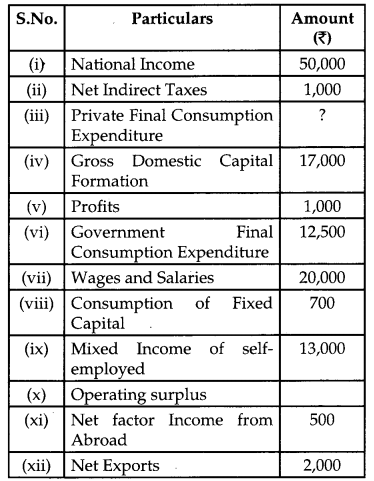Answer: Operating surplus = (i) – [(vii) + (ix) + (xi)] = 50,000 – (20,000 + 13,000 + 500) = ₹ 16,500 crores. Private final consumption expenditure = (i) – [(iv) + (vi) + (xi) + (xii)] + (viii) + (ii) = 50,000 – (17,000 + 12500 + 2000 + 500) + 700 + 1000 = ₹ 19,700 crores ### CBSE Previous Year Question Papers Class 12 Economics 2019 Delhi Set – III Note: Except for the following questions, all the remaining questions have been asked in 1 previous sets. Section – A Question 5. Good X and Good Y are complementary goods. ^ If price of Good X increases, discuss briefly its likely impact on the demand for Good Y.  OR If the income of a consumer increases, discuss briefly its likely impact on the demand for a normal good, Good X. Answer: Goods X and goods Y are complementary goods which are jointly demanded therefore if the price of good X increases the demand for good Y will decrease. This is due to the inverse relationship between the price of the given good and demand for it. OR If the income of a consumer increases, demand for a commodity rises. The consumer starts buying more good than before hence rightward shifting of demand curve will take place in this case. In the graph, original demand curve is DD but when income increases the demand for normal good increases and curve shifts from DD to D1D1.Question 7. Explain the law of equi-marginal utility.  OR State and discuss the conditions of consumer’s equilibrium under ordinal approach. Answer: Law of equi-Marginal Utility : The law was presented by an economist H.H. Gorsen. According to this law “A person can get maximum utility with his given income when it is spent on different commodities in such a way that the marginal utility of money spent on each item is equal”. Assumptions of the law : 1) There is no change in the prices of the goods. 2) The income of consumer is fixed. 3) The marginal utility of money is constant. 4) Consumer is normal person so he tries to seek maximum satisfaction. Explanation with schedule and diagram : Suppose consumer has six dollars that he want to spend on apples and bananas in order to obtain maximum total utility. The following table shows marginal utility (MU) of spending additional dollars of income on apples and bananas:  Money (Units) MU of apples MU of Bananas 1 10 8 2 9 7 3 8 6 4 7 5 5 6 4 6 5 3 The above schedule shows that consumer can spend six dollars in different ways : 1)$1 on apples and $5 on bananas. The TU he get is : The above schedule shows that consumer can [(10) + (8 + 7 + 6 + 5 + 4)] = 40 utils 2)$2 on apples and $4 on bananas. The TU he get is : [(10 + 9) + (8+ 7 + 6 + 5)] = 45 utils 3)$3 on apples and $3 on bananas. The TU he get is : [(10 + 9 + 8) + (8 + 7 + 6) ] = 48 utils 4)$4 on apples and $2 on bananas. The TU he get is : [(10 + 9 + 8 + 7) + (8 + 7) ] = 49 utils 5)$5 on apples and $1 on bananas. The TU he get is : [(10 + 9 + 8 + 7 + 6) + (8)] = 48 utils TU for consumer is 49 utils that is highest obtainable with expenditure of$4 on apples and \$2 on bananas. Here the condition, MU of apple = MU of banana i.e. 7 = 7 is also satisfied.
OR
Condition of consumer’s equilibrium under ordinal approach:
Suppose a consumer consumes only two commodities X and Y and prices are Px and Py respectively.
Conditions of equilibrium
A consumer will be in equilibrium when the following two conditions are satisfied.
Condition-1 : A consumer gets maximum satisfaction when ratios of MU of the commodities to their respective prices are equal. It implies that, consumer spends his income in such a way that utility gained from the last rupee spent on each commodity is equal.
Condition-2 : The law of diminishing marginal utility must operate. If MU does not fall, consumer will never stop its consumption of that good.

Question 10.
Elaborate three main features of monopolistic competition form of market. 
Monopolistic competition is market structure that has some features of Perfect competition and some features of monopoly. It refers to a market situation wherein there are large number of small firms/sellers selling differentiated but closely related products. Features of monopolistic competition :
(i) Large number of seller : Large number of sellers selling closely related but differentiated products. Each firm acts independently and has limited share of the market which leads to limited degree of monopoly over price of the product.
(ii) Product differentiation- Products are differentiated on the basis of brand name, color, size etc. These differentiated products are close substitute of each other. Since a group of buyers prefers the product of a particular producer, that producer enjoys some monopoly in the market.
(iii) Freedom of entry and exit to firms – There exist no barriers to entry or exit, as a result firms earn only normal profits in the long run.
OR

Question 18.
If in an economy : 
Change in initial Investment (ΔI) = ₹ 1,200 crores
Marginal Propensity to Save (MPS) = 0.2
Find the values of:
(a) Investment Multiplier (k)
(b) Change in final income (ΔY)
Change in initial investment (ΔI) = ₹ 1200 crores
Marginal propensity to save (MPS) = 0.2
(a) Investment multiplier (k) = $$\frac{1}{\mathrm{MPS}}=\frac{10}{2}$$ = 5

(b) Change in final income (ΔY) = ?
K = $$\frac{\Delta \mathrm{Y}}{\Delta \mathrm{I}}$$
ΔY = K × ΔI
= 5 × 1200 = ₹ 6000 crores

Question 21.
(a) How are tax receipts different from non-tax receipts? Discuss briefly. [3,1]
(b) State any two items of revenue expenditure in a Govenment budget.
(a) Difference between tax receipt and non-tax receipts

 Basis Tax Receipts Non-Tax Receipts Meaning Tax revenue in­cludes receipts from all types of taxes. Non tax revenue is the revenue of the government from sources other than taxes. Nature A tax is a legal and compulsory payment imposed by the government. It is not a compul­sory payment. Benefit No direct benefit is received in exchange of tax paid It is a paid in lieu of the benefit or services received. Examples Income tax, wealth tax, GST etc. Fees, fines, licence fee etc.

(b) Two items of revenue expenditure in the government budget are payment of wages and salaries and expenditure on education, health services, pensions, subsidies etc.

Question 22.
Given the following data, find the missing values of ‘Gross Domestic Capital Formation’ and ‘Wages and Salaries’.